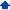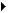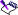### Formatting equation elements

Equation Editor lets you change the color and style of an equation element and apply a different style to a character that you add to an equation. You can also specify new size and font settings to change the default formatting of an equation.

#### To change the color of an equation element1 Select an equation in the document.
 2 Click Edit(Open) equation object.
 3 Click the Edit equation tab.
 4 Select an equation element.
 5 Click Color.
 6 In the Color dialog box, click a color on the palette.

#### To change the style of an equation element1 Select an equation in the document.
 2 Click Edit(Open) equation object.
 3 Click the Edit equation tab.
 4 Select an equation element.
 5 From the Style list box, choose one of the following:
 • Standard — lets you use the predefined style or font that conforms to mathematical typesetting conventions
 • Greek — lets you use Greek characters
 • Other — lets you define a style or font
 6 From the Size list box, choose one of the following:
 • Normal — lets you use the formatting of the paragraph that contains the equation
 • Subscript — lets you use the defined subscript size
 • Sub-subscript — lets you use the defined sub-subscript size
 • Symbol — lets you use the defined symbol size
 • Sub-symbol — lets you use the defined sub-symbol size
 • Other — lets you specify a size

#### To apply a different style to a character that you add to an equation1 Select an equation in the document.
 2 Click Edit(Open) equation object.
 3 Click the Edit equation tab.
 4 Click where you want to add a character in the equation.
 5 Press one of the following keyboard shortcuts:
 • Ctrl + G — assigns a Greek style
 • Ctrl + B — lets you specify a style (Other)

#### To change the default formatting of an equation1 Select an equation in the document.
 2 Click Edit(Open) equation object.
 3 Click the Font tab.
 4 Type a value in any of the following boxes:
 • Subscript/Superscript — lets you change the subscript and superscript sizes
 • Sub-subscript/Superscript — lets you change the sub-subscript and sub-superscript sizes
 • Symbol — lets you change the symbol size
 • Subsymbol — lets you change the sub-symbol size
 5 From the list boxes in the Font area, choose the font and style you want for the Greek lowercase, Greek uppercase, and Symbol characters.• If you specify a percentage for the font size setting, the font size adjusts to reflect any changes made to the font size of the paragraph that contains the equation.
 • Choose the Symbol font for symbol characters to ensure that they display correctly.